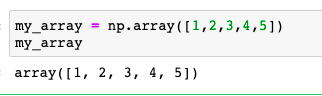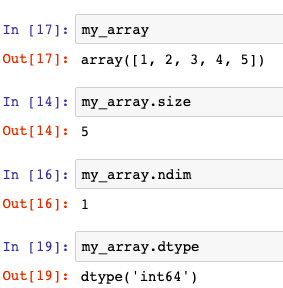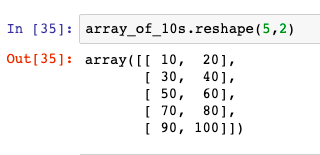Search
•Magdalena Konkiewicz

# A Gentle Intro to NumPyCredit to Pexels

The name NumPy stands for numerical python and has been designed to facilitate processing and mathematical operations of multidimensional arrays. It is an open-source library and many other scientific packages are based on it.

This article will walk through some of the NumPy basics such as creating arrays and performing simple operations on them.

Let’s get started

If NumPy is not installed in your environment you will need to install it with the following command:

```
pip install numpy

```

Once you have the package preinstalled you can start using with the following import:

```
import numpy as np

```

Creating arrays

Once you have imported the library you can start creating arrays. The simplest way to create arrays is by using lists:The code above creates a one-dimensional array with five elements.

You can check the details of the array using a shape attribute. Calling shape on our array confirms that the array is one dimensional and has five elements.The same way we created a one-dimensional array we can create any multidimensional array. Let’s create now a two-dimensional array as an example:As you can see you can use shape attribute to see array dimensions. Two-dimensional arrays can be thought of as matrices. The two-dimensional NumPy array above has three rows and five columns (3, 5).

Probably the most used dimensions of NumPy arrays that you will encounter will be one-dimensional array (standard array as in our first example) and two-dimensional array(the matrix style as in our second example). However, you could create any dimensional arrays using NumPy.

Basic array attributes

You already have met one of the NumPy array attributes called shape. The other important attributes will be size, ndim, and dtype. Let’s demonstrate its usage on our first array.The size attribute gives us a number of elements in the NumPy array, 5 in our case. The ndim confirms we have a one-dimensional array and dtype tells us that the elements are integers.

Similarly, we can check the attributes of the second example array that we have used:You can see that here we have 15 elements, 2 dimensions, and data again consists of integers.

Arange function

There is a convenient utility function to create NumPy arrays called arange(). The function takes three parameters: initial index (inclusive), end_index (exclusive) and step size to create arrays. Let’s demonstrate it here:As you can see here, we have created an array of numbers from 10 to 100 where each number is incremented by 10.

Reshape function

The other useful function to manipulate NumPy arrays is reshape() function. The function can be used to change array dimensions. Let’s apply it to our arra_of_10s:We have just reshaped one-dimensional array with ten elements to two-dimensional array with 5 rows and 2 columns.

The two functions mentioned in the last checkpoints arange() and reshape() are often used together to create artificial arrays and help us avoid typing long lists manually.

Mathematical operations on the arrays

Once created you can do the traditional mathematical operation to elements of NumPy array. Let’s try some multiplication and addition:We have just multiplied all element arrays by three and additionally added number three to each element.

Aggregate functions

Another useful operation that you can apply to NumPy arrays are aggregate functions. That way you can compute sums of all elements of the array, its minimum, and maximum value, or average of elements. Let’s see a real-life example:In the example above we have created our array of 10s and reshaped it to 2 row and 5 columns two-dimensional array. Then we have found sum, min, max and average values for the array. All of this with one line of code each.

This is the end of the short introduction to NumPy. I hoped you have enjoyed the walkthrough and you are now able to use some basic library functionality.